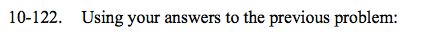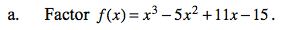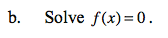Home > CCA2 > Chapter 10 > Lesson 10.2.2 > Problem10-122

10-122.
1. Using your answers to the previous problem: Homework Help ✎

1. Factor f(x) = x3 − 5x2 + 11x − 15.

2. Solve f(x) = 0.There are 2 factors. (x − 3) is one factor. Use long division to find the other factor.Once the polynomial is factored, use the Zero Product Property to solve.

This is a 3rd degree polynomial function, so find 3 answers. One of the answers is x = 1 + 2i.